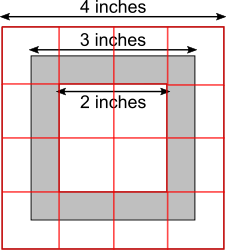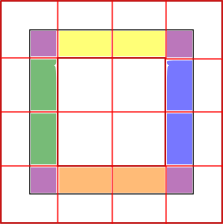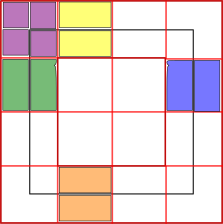#### You may also like### Hallway Borders

What are the possible dimensions of a rectangular hallway if the number of tiles around the perimeter is exactly half the total number of tiles?### Square Pegs

Which is a better fit, a square peg in a round hole or a round peg in a square hole?### Boxed In

A box has faces with areas 3, 12 and 25 square centimetres. What is the volume of the box?

# Nested Squares

##### Age 11 to 14 ShortChallenge Level

Splitting the diagram into one-inch squares
The largest square is 4 squares long, and the smallest square in the middle is 2 squares long.

Then, we can put the shaded pieces together to make whole square inches.So there are 5 shaded squares, out of 16 squares in total.

So the proportion of the area which is shaded is $\frac5{16}$.

Finding the areas of the squares
The whole diagram is a 4 inch by 4 inch square, so has area 4$\times$4 = 16 square inches.The shaded part is contained within a 3 by 3 square, so has area 3$\times$3 = 9 square inches.There is a hole in the shaded part, which is a 2 by 2 square. So the area of the hole is 2$\times$2 = 4 square inches.

So the area of the shaded part is
9$-$4 = 5 square inches.

So the proportion of the area which is shaded is $\frac5{16}$.

You can find more short problems, arranged by curriculum topic, in our short problems collection.